# Olympiad Test: Linear Equations In One Variable

## 20 Questions MCQ Test Mathematical Olympiad Class 8 | Olympiad Test: Linear Equations In One Variable

Description
Attempt Olympiad Test: Linear Equations In One Variable | 20 questions in 40 minutes | Mock test for Class 8 preparation | Free important questions MCQ to study Mathematical Olympiad Class 8 for Class 8 Exam | Download free PDF with solutions
QUESTION: 1

### A boat goes downstream and covers the distance between two ports in 4 hours, while it covers the same distance upstream in 5 hours. If the speed of the stream is 2km/hour. what is the speed of boat in still water ?

Solution:

Let the speed of boat in still water be x km/hour.
∴ (x + 2) 4 = (x – 2) 5
⇒  4x + 8 = 5x - 10
⇒ x = 18 km/hr

QUESTION: 2

### The ages of Mohan and Sohan are in the ratio 9 : 7. Ten years ago their ages were in the ratio 7 : 5. What is the difference between their present ages?

Solution:

Let Mohan’s age = 9x
and Sohan’s age = 7x
10, years ago,
Mohan’s age = 9x – 10
Sohan’s age = 7x – 10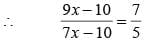⇒ 45x – 50 = 49x – 70
⇒ 4x = 20 ⇒ x = 5
∴ Mohan’s age = 9 × 5 = 45
and Sohan’s age = 7 × 5 = 35
Hence difference = 45 – 35 = 10 years

QUESTION: 3

### The sum of two numbers is 360. If 65% of one number is equal to 85% of the other. What is the largest number among them?

Solution:

Let the one number be x.
Other number = 360 – x
Now 65% of x = 85% of (360 – x)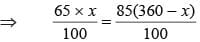⇒ 65 x + 85x = 85 × 360
⇒ 150x = 85 × 360
⇒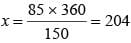QUESTION: 4

A certain number of workers can finish a piece of work in 70 days. If there are 20 men less, it would take 10 days more for the same work to be finished. How many workers were there in starting?

Solution:

Let the no. of workers in starting be x.
∴ 70 × x = (x – 20) 80
⇒ 70 x = 80x – 20 × 80
⇒ 10x = 20 × 80
⇒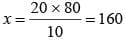QUESTION: 5

The sum of three consecutive multiples of 11 is 363. Which of these multiple is greatest?

Solution:

Let the numbers be
11x, 11(x + 1), 11(x + 2)
Hence 11x + 11(x + 1) + 11(x + 2) = 363
⇒ 11x + 11x + 11 + 11x + 22 = 363
⇒ 33x + 33 = 363
⇒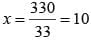Greatest multiple = 11(x + 2)
= 11 (10 + 2) = 11 × 12 = 132

QUESTION: 6

Arun’s age is three times his son’s age. 10 years age he was 5 times his son’s age. What is the sum of their present ages?

Solution:

Let Son’s age = x years
∴ Arun’s age = 3x.
Now 10 years ago, son’s age = x – 10.
Given Arun’s age = 3x – 10
⇒ 3x -10 = 5 (x – 10)
⇒ 3x – 10 = 5x – 50
2x = 40 ⇒ x = 20 years
Arun’s  age = 3 × 20 = 60 years
Sum of Arun’s and Son’s age = 20 + 60
= 80 years

QUESTION: 7

Nirmal thinks of a number and subtracts 5/2 from it. He multiplies the result by 8. The result now obtained is 3 times the same number he thought of. Find the number.

Solution:

Let the number be x.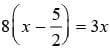⇒ 8x - 20 = 3x
⇒ 5x = 20
⇒ x = 4

QUESTION: 8

The difference between the digits of a two-digit number is 3. If the digits are interchanged and the resulting number is added to the original number we get 143. What was the original number?

Solution:

Let the unit’s digit be x.
Ten’s digit = x + 3.Original number =10(x + 3) + 1(x)
= 10x + 30 + x
= 11x + 30
After interchange, resulting number
= 10 (x) + 1 (x + 3)
= 10x + x + 3
= 11x + 3
∴ 11x + 3 + 11x + 30 = 143
⇒ 22x + 33 = 143
⇒ 22x = 143 – 33
⇒ 22x = 110
⇒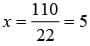Original number = 11x + 3 = 11 × 5 + 3
= 55 + 3 = 58

QUESTION: 9

A grand father is ten times older than his grandson. He is then also 54 years older than him. What is the difference of their present ages?

Solution:

Let the age of grand son be x years.
Grand father’s age = 10x.
⇒ 10x = 54 + x
⇒ 9x = 54
⇒ x = 6
Grand father’s age = 10 × 6 = 60 years
Difference of their present ages = 60 – 6 = 54.

QUESTION: 10

An altitude of a triangle is five-thirds the length of its corresponding base. If the altitude is increased by 4 cm and the base is decreased by 2 cm, but the area of triangle remains the same. Find the base and altitude of the triangle respectively.

Solution:

Let the base be x
Altitude -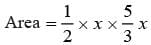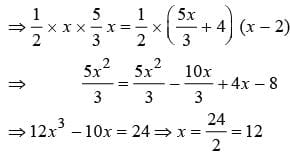∴ Altitude = 12 × 5/3 = 20 cm

QUESTION: 11

A field can be ploughed in 18 days. If everyday an additional area of 16 hectares is ploughed, the field can be ploughed in 12 days. What is the area of the field?

Solution:

Let the area of the field ploughed daily be x hectares.
Area of the field = 18x
⇒ 18x = (x + 16) 12
⇒ 18x = 12x + 16 × 12
⇒ 6x = 16 × 12
⇒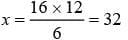​Area of the field = 18 × 32 = 576 hectares

QUESTION: 12

The sum of the digits of a two number is 9. If 9 is subtracted from the number its digits are interchanged. What is the number?

Solution:

Let the unit’s digit be x.
Ten’s digit = 9 – x
Number = 10 (9 – x) + 1(x)
= 90 – 10x + x= 90 – 9x
90 – 9x – 9 = x(10) + 1(9 – x)
⇒ 81 – 9x = 10x + 9 – x
⇒ 81 – 9x = 9x + 9
⇒ 18x = 72 ⇒ x = 4
Number = 90 – 9 × 4 = 90 – 36 = 54

QUESTION: 13

What is the value of x in the given equatiom?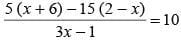Solution: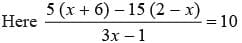⇒ 5x + 30 – 30 + 15x = 30x – 10
⇒ 20x + 10 = 30x
⇒ 10x = 10 ⇒ x = 1

QUESTION: 14

What is the value of x in the given equation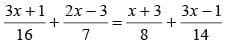Solution: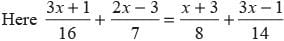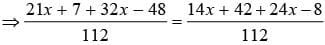⇒ 53x - 41 = 38x + 34
⇒ 15x = 34 + 41
⇒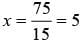QUESTION: 15

What is the value of x if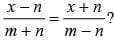Solution:

⇒ (x – n) (m – n) = (x + n)(m + n)
⇒ mx – mn – nx + n2 = mx + mn + nx + n2
⇒ - mn – nx = mn + nx
⇒ 2nx = -2mn
⇒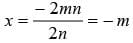QUESTION: 16

A shirt is sold for Rs. 1498 and the seller gains 7% on it. What is the cost price of the shirt ?

Solution:

Let the cost price of the shirt be Rs. x.
∴ x + 7% of x = 1498
⇒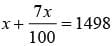⇒ 107x 1498 x 100
⇒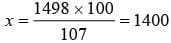QUESTION: 17

Half of a herd of deer are grazing in the field and three fourth of remaining are playing nearby. The rest nine are drinking water from the river. What is the number of deer in the herd?

Solution:

Let number of deer be x.
∴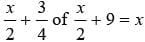⇒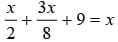⇒ 4x + 3x 72 = 8x
⇒  x = 72

QUESTION: 18

The ages of Raju and Rajan are in the ratio 5 : 8. If Raju was 5 years older and Rajan 4 years younger, the age of Raju would have been the same as the age of Rajan. What is the age of Raju?

Solution:

Let Raju’s age = 5x.
∴ Rajan ‘s age = 8x
∴ 5x + 5 = 8x – 4
⇒ 3x = 9 ⇒ x = 3
Raju’s age = 5 × = 15 years

QUESTION: 19

If 1/2 is subtracted from a number and the difference is multiplied by 8, the result is 12. What is the number?

Solution:

Let the number be x.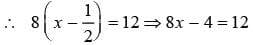⇒ 8x = 16
⇒ x = 2

QUESTION: 20

Mohaesh travelled 1/8 th of his Journey by bus, 1/4 th by taxi, 3/5 th by train and remaining 8 km by foot. What is the length of his total Journey?​

Solution:

Let length of total Journey be x km.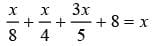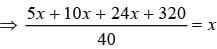⇒ 39x + 320 = 40x
⇒ x = 320Use Code STAYHOME200 and get INR 200 additional OFF Use Coupon Code